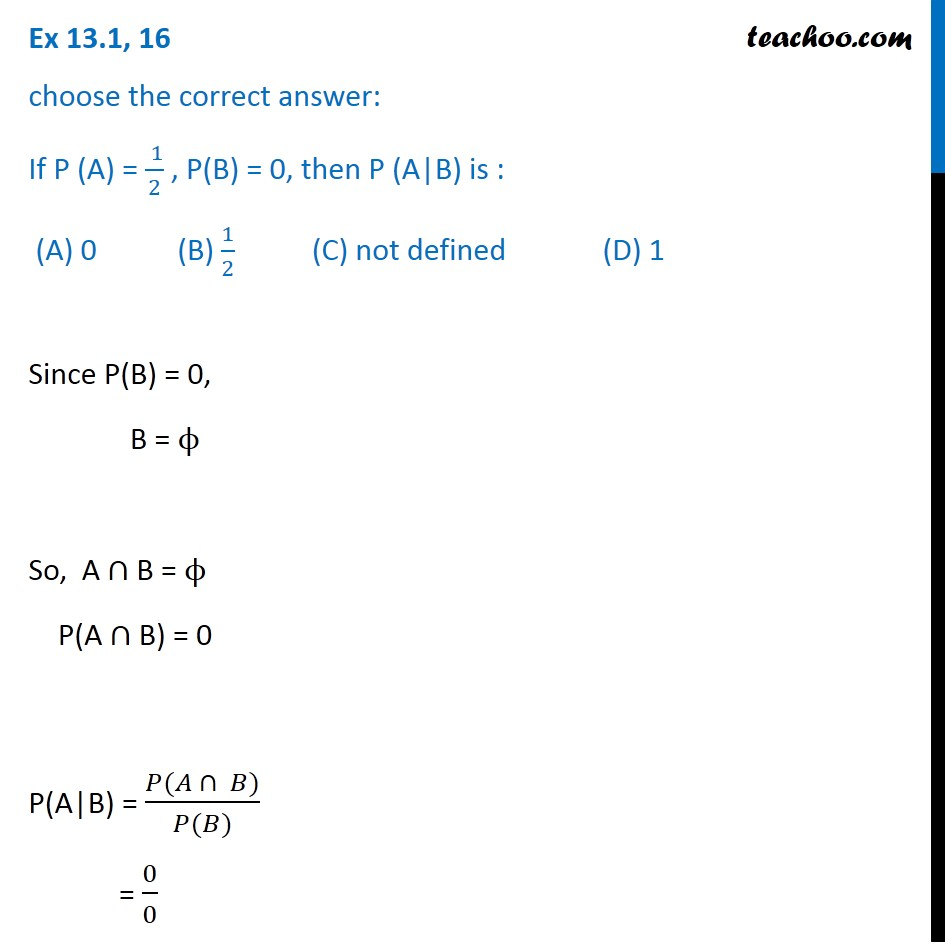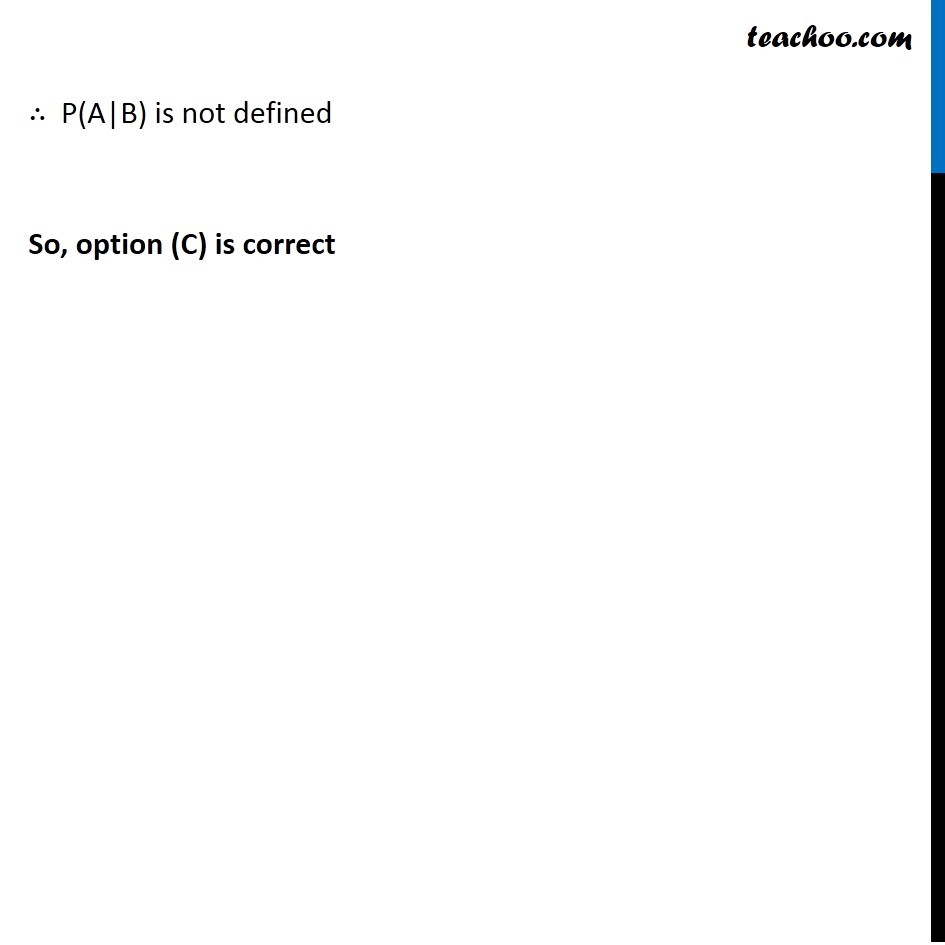Conditional Probability - Values given

Chapter 13 Class 12 Probability
Concept wiseIntroducing your new favourite teacher - Teachoo Black, at only ₹83 per month

### Transcript

Ex 13.1, 16 choose the correct answer: If P (A) = ( 1)/2 , P(B) = 0, then P (A|B) is : (A) 0 (B) 1/2 (C) not defined (D) 1Since P(B) = 0, B = ϕ So, A ∩ B = ϕ P(A ∩ B) = 0 P(A|B) = (𝑃(𝐴 ∩ 𝐵))/(𝑃(𝐵)) = 0/0 ∴ P(A|B) is not defined So, option (C) is correct